# Evomark grammars

• Container
• Inline command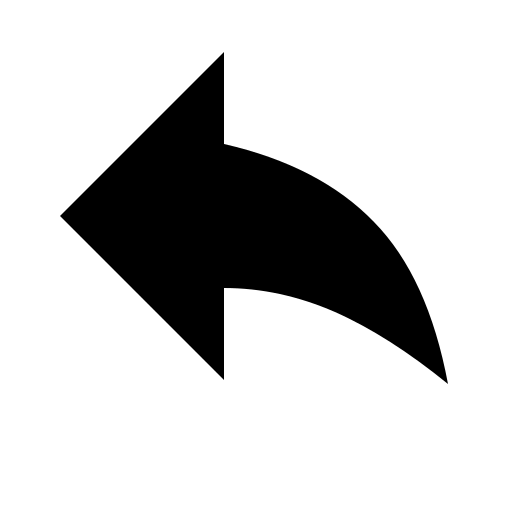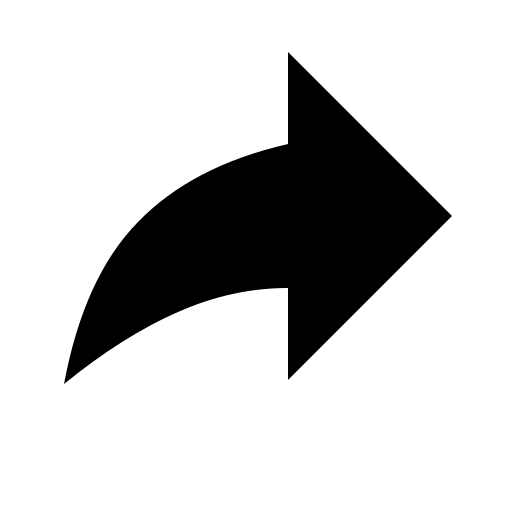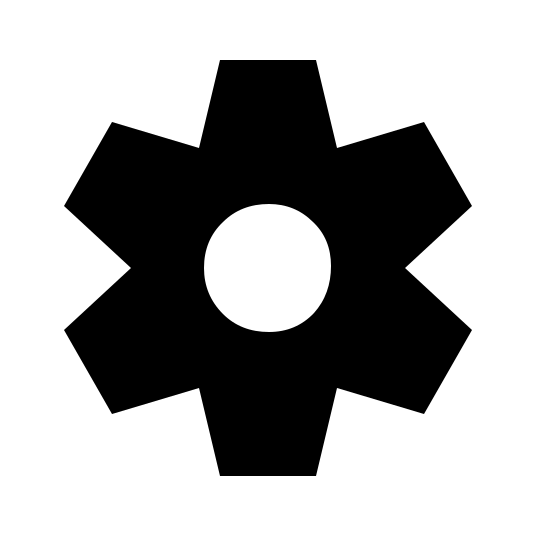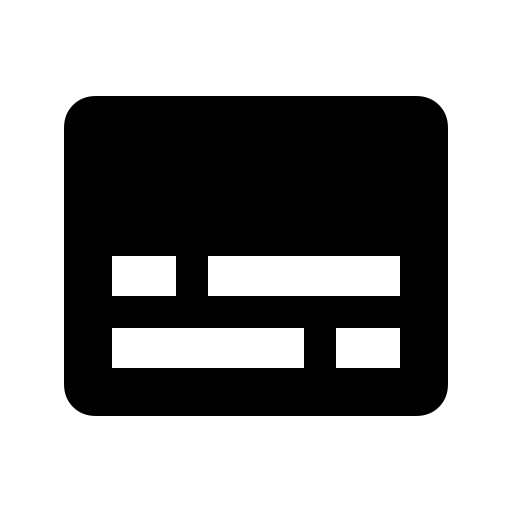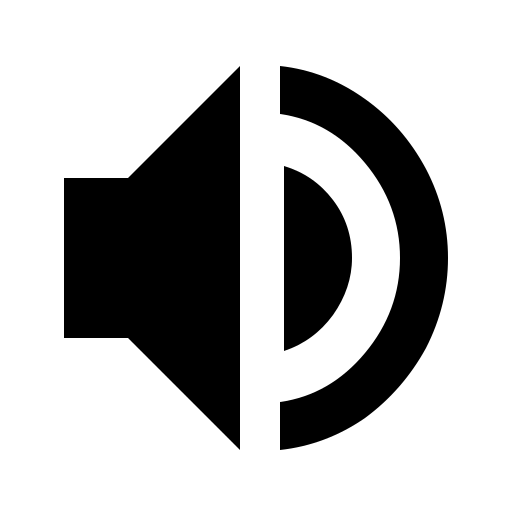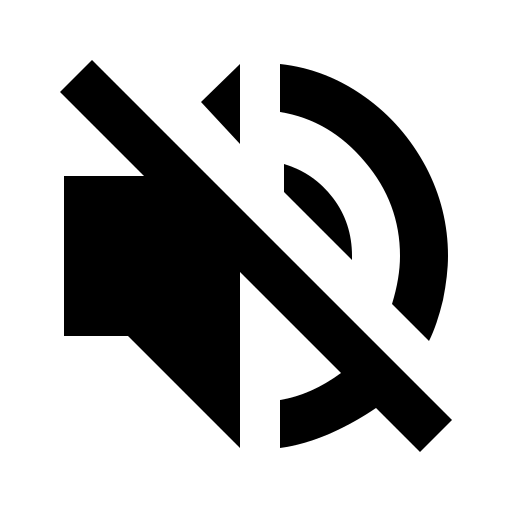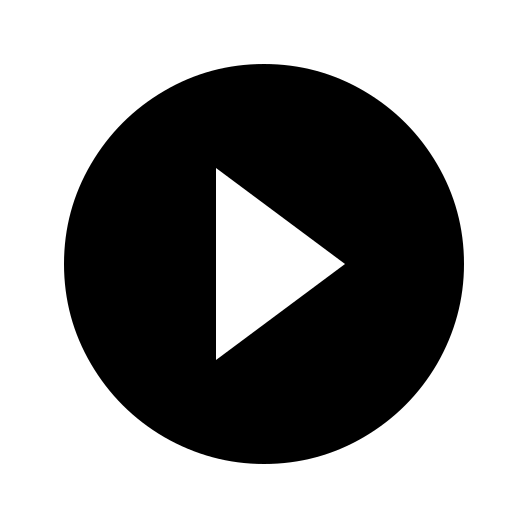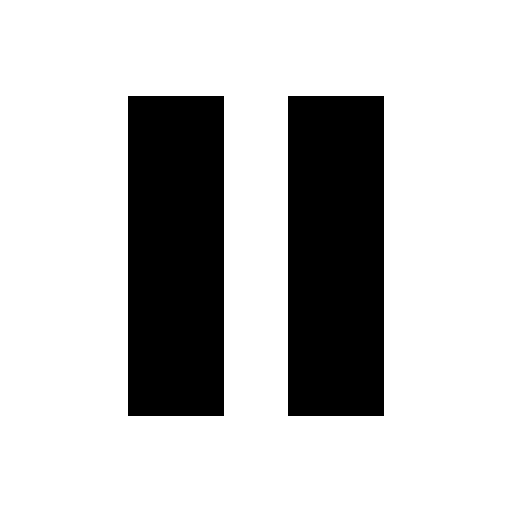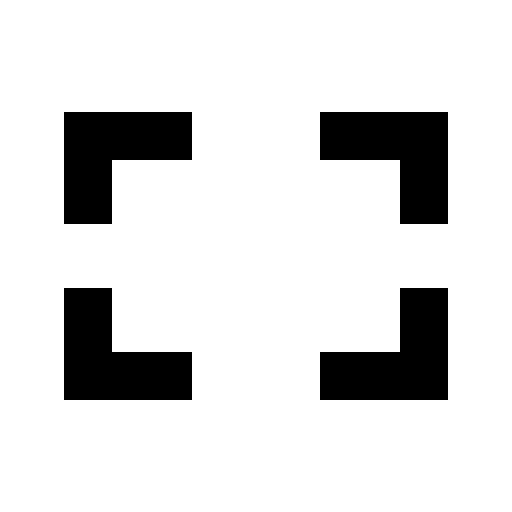# Container delimiter with direction (Important)

Evomark supports container delimiters to be the same for opener and closer. However, in some cases you may want to specify it in order to avoid using too long delimiters.
• ==> is equivalent to === opener.
• ==| is equivalent to === closer.
Children containers can have an opener with the same length to their parents using ==> and ==|. Here we give a example.
==> Box
==> Box
I'm allowed.
==|
==|
In contrast, the following code will give out errors.
=== Box
=== Box
I will give out a error.
===
===

# Container examples

## Figure

=== Figure {src:"Irena.jpg"}
This is Irena.
===This is Irena.

## Proof

=== Proof
We know that $1+2=3$ and $2+1=3$. Therefore $1+2=2+1$.
===
We know that and . Therefore .

## Table

You can put TOML inside the Table container.
==== Table
[[item]]
Name = "Mike"
Subject = "Math"
[[item]]
Name = "Sarah"
Subject = "English"
~~~~
You can put the caption after the fence
====
Here is the effect.
Table 1
 Name Subject Grade Mike Math 99 Sarah English 100
You can put the caption after the fence
You can also use src attribute to specify a .toml/.yml/.json file. The file should describe a dictionary with the following structure
{
item:[
{
Key1: Value1_1
Key2: Value1_2
...
},
{
Key1: Value2_1
Key2: Value2_2
...
}
...
]
}
javascript

## Code & Styled Box

==== Code
=== Box {style:"text-align:center;background-color:green;color:red;"}
I'm a box produced by the code above.
===
====
=== Box {style:"text-align:center;background-color:blue;color:red;"}
I'm a box produced by the code above.
===
I'm a box produced by the code above.